# Harmonic Fields

This continues from the optical fields and Maxwell's equations tutorial.

Optical fields are harmonic fields that vary sinusoidally with time. The field vectors defined in the preceding tutorials are all real quantities. For harmonic fields, it is always convenient to use complex fields. We define the space- and time-dependent complex electric field, E(r, t), through its relation to the real electric field, E(r, t):

E(r, t) = E(r, t) + E*(r, t) = E(r, t) + c.c.,                (39)

where c.c. means the complex conjugate. In our convention, E(r, t)  contains the complex field components that vary with time as exp(-iωt) with positive values of ω, while E*(r, t) contains those varying with time as exp(iωt) with positive ω, or exp(-iωt) with negative ω. The complex fields of other field quantities are similarly defined.

With this definition for the complex fields, all of the linear field equations retain their forms. In particular, Maxwell's equations for the complex optical fields are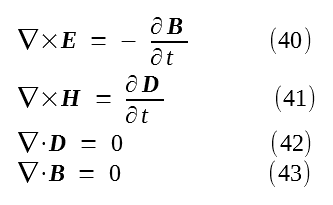The wave equation in terms of the complex electric field iswhile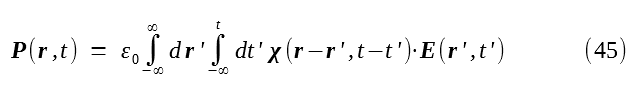andIt is important to note that while P, D, and E are complex, χ(r - r', t - t') and ε(r - r', t - t') in (45) and (46) are always real.

For a harmonic optical field of wavevector k and angular frequency ω, its complex electric field can be further written as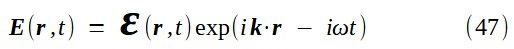where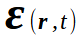is the space- and time-varying field envelope, such as that for a modulated field, a guided field, or an optical pulse. Other complex quantities, such as, can be similarly expressed. The phase factor in (47) indicates the direction of wave propagation: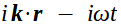,   forward propagating in k direction;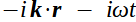,  backward propagating in -k direction.

The light intensity, or irradiance, is the power density of the harmonic optical field. It can be calculated by time averaging of the Poynting vector over one wave cycle:where Re() means taking the real part. We can define a complex Poynting vector:

S = E x H*                                    (49)

so thatwhich has the same form as the relation between the real and complex fields defined in (39) except that the real Poynting vector in this relation is time averaged. The light intensity, I, is simply the magnitude of the real time-averated Poynting vector: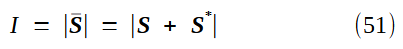where I is in watts per square meter.

For harmonic optical fields, it is often useful to consider the complex fields in the momentum space and frequency domain defined by the following Fourier-transform relations:Note that E(k, ω) in (52) is defined for ω > 0 only, and the integral for the time dependence of E(r, t) in (53) extends only over positive values of ω. This is in accordance with the convention we used to define the complex field E(r, t) in (39). All other space- and time-dependent quantities, including other field vectors and the permittivity and susceptibility tensors, are transformed in a similar manner. Through the Fourier transform, the convolution integrals in real space and time become simple products in the momentum space and frequency domain. Consequently, we haveThe next part continues with the linear optical susceptibility tutorial.